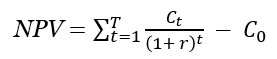## IRR Calculator

Calculate the IRR of your investment quickly and easily with this online calculator. Simply input the investment size and cash flow per period to determine the IRR, as well as the gross return and net cash flow.

IRR Calculator

## Using the IRR calculator

The IRR calculation tool is user-friendly: enter the initial investment amount (in any currency, e.g. USD, EUR, CHF) and the number of periods (up to 25) for which you want to analyze the cash flow. Then, input the cash flow for each period (past or forecasted).

Once ready, press “Calculate” and our Internal Rate of Return calculator will output:

• the Internal Rate of Return, a.k.a. discount rate
• the Gross Return in percentages
• the Net Cash Flow (Profit – Loss)

## What is Internal Rate of Return?

The Internal Rate of Return (IRR) is a measure of the profitability of an investment and is defined as the discount rate that makes the net present value (NPV) of all cash flows from a project equal to zero. It is also referred to as the “economic rate of return” and “discounted cash flow rate of return.” The term “Internal” in the name implies that external factors such as capital cost and currency inflation are not taken into account. IRR gives more weight to earlier cash flows, reflecting the time preference of investors.

IRR is commonly used to evaluate the potential profitability of investments, with a higher IRR indicating a more desirable project and a lower IRR indicating a more risky and less desirable project. It is often used to rank prospective projects on a relatively even basis. However, it’s important to note that comparing IRR across projects of different expected lengths can be misleading. Additionally, IRR doesn’t take into account reinvestment rates, which are more directly tied to the capital cost. For cases when cost of capital needs to be considered, the modified internal rate of return (MIRR) is often used as an alternative. It’s recommended to use IRR in conjunction with other metrics such as NPV.

## IRR formula

Calculating the Internal Rate of Return (IRR) can be done programmatically or by using tools such as an IRR calculator. There is no analytical solution to this issue and it is based on the Net Present Value (NPV) formula.where r is the discount rate / interest rate and t is the number of cash flow periods, C0 is the initial investment while Ct is the return during period t. One needs to replace NPV with zero and solve for r, for which there is no analytical solution since one can’t single out r in one side of the equation. For this reason, our calculator performs a recursive search until it finds a value of r which results in an NPV close to zero.

## IRR calculation example

In order to determine the Internal Rate of Return (IRR) for a given investment scenario, one can examine the relationship between the present value and the discount rate (or cost of capital).

For example, consider a project that requires an initial investment of \$10,000 and is expected to return \$15,000 in three years time with positive cash flows in each year of \$3,800, \$4,400, and \$6,800 respectively. To calculate the IRR for this scenario, we need to find the discount rate (r) at which the net present value (NPV) of all cash flows is equal to zero.

One way to solve this problem is by graphically examining the relationship between the present value and the discount rate. This can be done by plotting the NPV of the cash flows at various discount rates and identifying the point where the NPV equals zero. This point represents the IRR for the investment scenario.

Scroll to Top